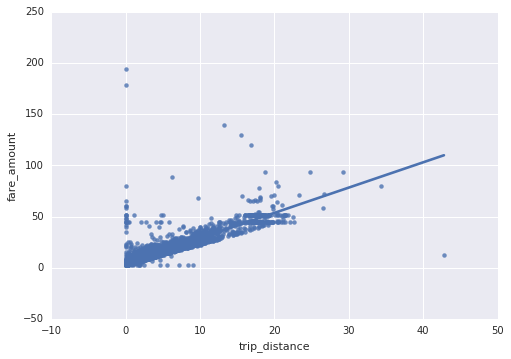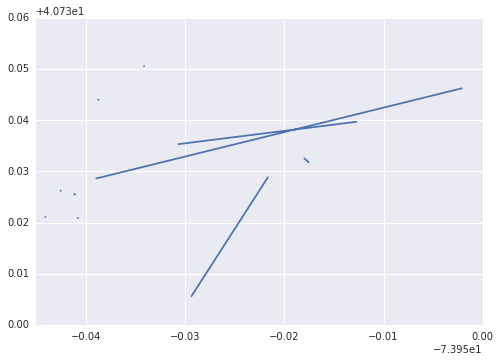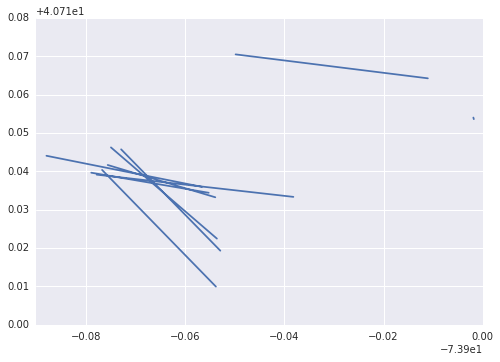# Explore and create ML datasets

In this notebook, we will explore data corresponding to taxi rides in New York City to build a Machine Learning model in support of a fare-estimation tool. The idea is to suggest a likely fare to taxi riders so that they are not surprised, and so that they can protest if the charge is much higher than expected.

Let's start off with the Python imports that we need.

In :
from google.cloud import bigquery
import seaborn as sns
import pandas as pd
import numpy as np
import shutil


### Extract sample data from BigQuery

The dataset that we will use is a BigQuery public dataset. Click on the link, and look at the column names. Switch to the Details tab to verify that the number of records is one billion, and then switch to the Preview tab to look at a few rows.

Write a SQL query to pick up the following fields

  pickup_datetime,
pickup_longitude, pickup_latitude,
dropoff_longitude, dropoff_latitude,
passenger_count,
trip_distance,
tolls_amount,
fare_amount,
total_amount

from the dataset and explore a random subsample of the data. Sample size should be about 10,000 records. Make sure to pick a repeatable subset of the data so that if someone reruns this notebook, they will get the same results.

Hint (highlight to see)

Set the query string to be:
SELECT above_fields FROM
nyc-tlc.yellow.trips
WHERE
ABS(MOD(FARM_FINGERPRINT(CAST(pickup_datetime AS STRING)), 100000)) = 1
Then, use the BQ library:
trips = bigquery.Client().query(query).execute().result().to_dataframe()

In :
# TODO: write a BigQuery query for the above fields
# Store it into a Pandas dataframe named "trips" that contains about 10,000 records.


### Exploring data

Let's explore this dataset and clean it up as necessary. We'll use the Python Seaborn package to visualize graphs and Pandas to do the slicing and filtering.

In :
ax = sns.regplot(x = "trip_distance", y = "fare_amount", ci = None, truncate = True, data = trips)Hmm ... do you see something wrong with the data that needs addressing?

It appears that we have a lot of invalid data that is being coded as zero distance and some fare amounts that are definitely illegitimate. Let's remove them from our analysis. We can do this by modifying the BigQuery query to keep only trips longer than zero miles and fare amounts that are at least the minimum cab fare ($2.50). What's up with the streaks at \$45 and \$50? Those are fixed-amount rides from JFK and La Guardia airports into anywhere in Manhattan, i.e. to be expected. Let's list the data to make sure the values look reasonable. Let's examine whether the toll amount is captured in the total amount. In : tollrides = trips[trips['tolls_amount'] > 0] tollrides[tollrides['pickup_datetime'] == '2014-05-20 23:09:00']  Out: pickup_datetime pickup_longitude pickup_latitude dropoff_longitude dropoff_latitude passenger_count trip_distance tolls_amount fare_amount total_amount 2842 2014-05-20 23:09:00 -73.975610 40.751660 -73.953920 40.743240 1 2.22 5.33 8.5 14.83 4055 2014-05-20 23:09:00 -73.987960 40.754062 -73.956627 40.745962 2 3.11 5.33 11.5 21.30 5045 2014-05-20 23:09:00 0.000000 0.000000 0.000000 0.000000 1 6.38 5.33 20.0 26.33 5145 2014-05-20 23:09:00 -73.873527 40.773922 -73.944947 40.828902 5 8.35 5.33 25.0 36.33 5249 2014-05-20 23:09:00 -74.005625 40.736770 -73.919840 40.877687 1 14.33 2.44 42.0 54.43 5300 2014-05-20 23:09:00 -73.783898 40.648660 -73.981845 40.762872 6 18.18 5.33 52.0 69.30 5402 2014-05-20 23:09:00 -73.781987 40.644755 -73.921672 40.828412 1 19.08 5.33 50.5 56.83 5854 2014-05-20 23:09:00 -73.873210 40.774042 -73.978013 40.755800 5 10.45 5.33 30.5 36.83 5855 2014-05-20 23:09:00 -73.870160 40.760742 -73.980912 40.788492 4 10.37 5.33 30.5 45.91 5861 2014-05-20 23:09:00 -73.874445 40.774037 -73.982382 40.784930 1 9.97 5.33 30.5 44.10 5873 2014-05-20 23:09:00 -73.984350 40.761760 -73.859030 40.718510 2 10.04 5.33 31.5 37.83 5896 2014-05-20 23:09:00 -73.874567 40.774102 -73.986480 40.759202 1 11.43 5.33 37.0 54.04 5919 2014-05-20 23:09:00 -74.011087 40.724797 -74.026347 40.750997 1 4.09 11.00 45.0 61.00 Looking a few samples above, it should be clear that the total amount reflects fare amount, toll and tip somewhat arbitrarily -- this is because when customers pay cash, the tip is not known. So, we'll use the sum of fare_amount + tolls_amount as what needs to be predicted. Tips are discretionary and do not have to be included in our fare estimation tool. Let's also look at the distribution of values within the columns. In : trips.describe()  Out: pickup_longitude pickup_latitude dropoff_longitude dropoff_latitude passenger_count trip_distance tolls_amount fare_amount total_amount count 10789.000000 10789.000000 10789.000000 10789.000000 10789.000000 10789.000000 10789.000000 10789.000000 10789.000000 mean -72.577466 39.988518 -72.577056 39.991694 1.648716 2.837068 0.228359 11.165192 13.280564 std 10.071200 5.523964 10.068592 5.510504 1.282589 3.319036 1.144977 9.444876 11.267704 min -75.336579 0.000000 -75.381754 0.000000 0.000000 0.000000 0.000000 2.500000 2.500000 25% -73.992172 40.735837 -73.991581 40.734267 1.000000 1.010000 0.000000 6.000000 7.200000 50% -73.981871 40.753200 -73.980401 40.752872 1.000000 1.750000 0.000000 8.500000 10.000000 75% -73.967330 40.767309 -73.964102 40.767484 2.000000 3.110000 0.000000 12.500000 14.600000 max 0.000000 41.366138 0.000000 41.366138 6.000000 42.800000 16.000000 194.750000 204.750000 Hmm ... The min, max of longitude look strange. Finally, let's actually look at the start and end of a few of the trips. In : def showrides(df, numlines): import matplotlib.pyplot as plt lats = [] lons = [] goodrows = df[df['pickup_longitude'] < -70] for iter, row in goodrows[:numlines].iterrows(): lons.append(row['pickup_longitude']) lons.append(row['dropoff_longitude']) lons.append(None) lats.append(row['pickup_latitude']) lats.append(row['dropoff_latitude']) lats.append(None) sns.set_style("darkgrid") plt.plot(lons, lats) showrides(trips, 10)In : showrides(tollrides, 10)As you'd expect, rides that involve a toll are longer than the typical ride. ### Quality control and other preprocessing We need to do some clean-up of the data: 1. New York city longitudes are around -74 and latitudes are around 41. 2. We shouldn't have zero passengers. 3. Clean up the total_amount column to reflect only fare_amount and tolls_amount, and then remove those two columns. 4. Before the ride starts, we'll know the pickup and dropoff locations, but not the trip distance (that depends on the route taken), so remove it from the ML dataset 5. Discard the timestamp Let's change the BigQuery query appropriately. In production, we'll have to carry out the same preprocessing on the real-time input data. In : def sample_between(a, b): basequery = """ SELECT (tolls_amount + fare_amount) AS fare_amount, pickup_longitude AS pickuplon, pickup_latitude AS pickuplat, dropoff_longitude AS dropofflon, dropoff_latitude AS dropofflat, passenger_count*1.0 AS passengers FROM nyc-tlc.yellow.trips WHERE trip_distance > 0 AND fare_amount >= 2.5 AND pickup_longitude > -78 AND pickup_longitude < -70 AND dropoff_longitude > -78 AND dropoff_longitude < -70 AND pickup_latitude > 37 AND pickup_latitude < 45 AND dropoff_latitude > 37 AND dropoff_latitude < 45 AND passenger_count > 0 """ sampler = "AND ABS(MOD(FARM_FINGERPRINT(CAST(pickup_datetime AS STRING)), EVERY_N)) = 1" sampler2 = "AND {0} >= {1}\n AND {0} < {2}".format( "ABS(MOD(FARM_FINGERPRINT(CAST(pickup_datetime AS STRING)), EVERY_N * 100))", "(EVERY_N * {})".format(a), "(EVERY_N * {})".format(b) ) return "{}\n{}\n{}".format(basequery, sampler, sampler2) def create_query(phase, EVERY_N): """Phase: train (70%) valid (15%) or test (15%)""" query = "" if phase == 'train': # Training query = sample_between(0, 70) elif phase == 'valid': # Validation query = sample_between(70, 85) else: # Test query = sample_between(85, 100) return query.replace("EVERY_N", str(EVERY_N))  In [ ]: # TODO: try out train, test and valid here print (create_query('train', 100000))  In : def to_csv(df, filename): outdf = df.copy(deep = False) outdf.loc[:, 'key'] = np.arange(0, len(outdf)) # rownumber as key # Reorder columns so that target is first column cols = outdf.columns.tolist() cols.remove('fare_amount') cols.insert(0, 'fare_amount') print (cols) # new order of columns outdf = outdf[cols] outdf.to_csv(filename, header = False, index_label = False, index = False) print ("Wrote {} to {}".format(len(outdf), filename)) for phase in ['train', 'valid', 'test']: query = create_query(phase, 100000) df = bigquery.Client().query(query).to_dataframe() to_csv(df, 'taxi-{}.csv'.format(phase))  ['fare_amount', u'pickuplon', u'pickuplat', u'dropofflon', u'dropofflat', u'passengers', 'key'] Wrote 7645 to taxi-train.csv ['fare_amount', u'pickuplon', u'pickuplat', u'dropofflon', u'dropofflat', u'passengers', 'key'] Wrote 1814 to taxi-valid.csv ['fare_amount', u'pickuplon', u'pickuplat', u'dropofflon', u'dropofflat', u'passengers', 'key'] Wrote 1017 to taxi-test.csv  ### Verify that datasets exist In : !ls -l *.csv  -rw-r--r-- 1 root root 56326 Aug 30 21:32 taxi-test.csv -rw-r--r-- 1 root root 425723 Aug 30 21:32 taxi-train.csv -rw-r--r-- 1 root root 100355 Aug 30 21:32 taxi-valid.csv  We have 3 .csv files corresponding to train, valid, test. The ratio of file-sizes correspond to our split of the data. In : !head taxi-train.csv  2.5,-73.988954,40.758612,-73.952118,40.776227,2.0,0 2.5,-73.971783,40.763727,-73.971769,40.763768,2.0,1 3.0,-73.976258,40.786085,-73.974417,40.788517,2.0,2 3.0,-73.990085,40.746602,-73.993129,40.74765,2.0,3 3.5,-73.981335,40.781372,-73.981517,40.78386,2.0,4 3.5,-73.982673,40.727202,-73.978255,40.729524,2.0,5 3.5,-73.98582,40.744768,-73.978803,40.752505,2.0,6 3.5,-73.984404,40.767969,-73.988457,40.764324,2.0,7 3.5,-73.961372,40.769257,-73.966002,40.768292,2.0,8 3.5,-73.992835,40.768267,-73.93862,40.788212,2.0,9  Looks good! We now have our ML datasets and are ready to train ML models, validate them and evaluate them. ### Benchmark Before we start building complex ML models, it is a good idea to come up with a very simple model and use that as a benchmark. My model is going to be to simply divide the mean fare_amount by the mean trip_distance to come up with a rate and use that to predict. Let's compute the RMSE of such a model. In : from google.cloud import bigquery import pandas as pd import numpy as np import shutil def distance_between(lat1, lon1, lat2, lon2): # Haversine formula to compute distance "as the crow flies". Taxis can't fly of course. dist = np.degrees(np.arccos(np.sin(np.radians(lat1)) * np.sin(np.radians(lat2)) + np.cos(np.radians(lat1)) * np.cos(np.radians(lat2)) * np.cos(np.radians(lon2 - lon1)))) * 60 * 1.515 * 1.609344 return dist def estimate_distance(df): return distance_between(df['pickuplat'], df['pickuplon'], df['dropofflat'], df['dropofflon']) def compute_rmse(actual, predicted): return np.sqrt(np.mean((actual - predicted)**2)) def print_rmse(df, rate, name): print ("{1} RMSE = {0}".format(compute_rmse(df['fare_amount'], rate * estimate_distance(df)), name)) FEATURES = ['pickuplon','pickuplat','dropofflon','dropofflat','passengers'] TARGET = 'fare_amount' columns = list([TARGET]) columns.extend(FEATURES) # in CSV, target is the first column, after the features columns.append('key') df_train = pd.read_csv('taxi-train.csv', header = None, names = columns) df_valid = pd.read_csv('taxi-valid.csv', header = None, names = columns) df_test = pd.read_csv('taxi-test.csv', header = None, names = columns) rate = df_train['fare_amount'].mean() / estimate_distance(df_train).mean() print ("Rate =${0}/km".format(rate))
print_rmse(df_train, rate, 'Train')
print_rmse(df_valid, rate, 'Valid')
print_rmse(df_test, rate, 'Test')

Rate = $2.61647175325/km Train RMSE = 7.45944982351 Valid RMSE = 9.35408256465 Test RMSE = 5.44470804972  /usr/local/lib/python2.7/dist-packages/ipykernel/__main__.py:8: RuntimeWarning: invalid value encountered in arccos  The simple distance-based rule gives us a RMSE of$9.35 on the validation dataset. We have to beat this, of course, but you will find that simple rules of thumb like this can be surprisingly difficult to beat. You don't wnat to set a goal on the test dataset because you want to change the architecture of the network etc. to get the best validation error. Then, you can evaluate ONCE on the test data.

## Challenge Exercise¶

Let's say that you want to predict whether a Stackoverflow question will be acceptably answered. Using this public dataset of questions, create a machine learning dataset that you can use for classification.

What is a reasonable benchmark for this problem? What features might be useful?

If you got the above easily, try this harder problem: you want to predict whether a question will be acceptably answered within 2 days. How would you create the dataset?

Hint (highlight to see):

You will need to do a SQL join with the table of [answers]( https://bigquery.cloud.google.com/table/bigquery-public-data:stackoverflow.posts_answers) to determine whether the answer was within 2 days.